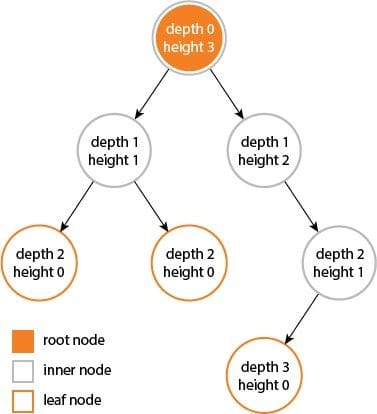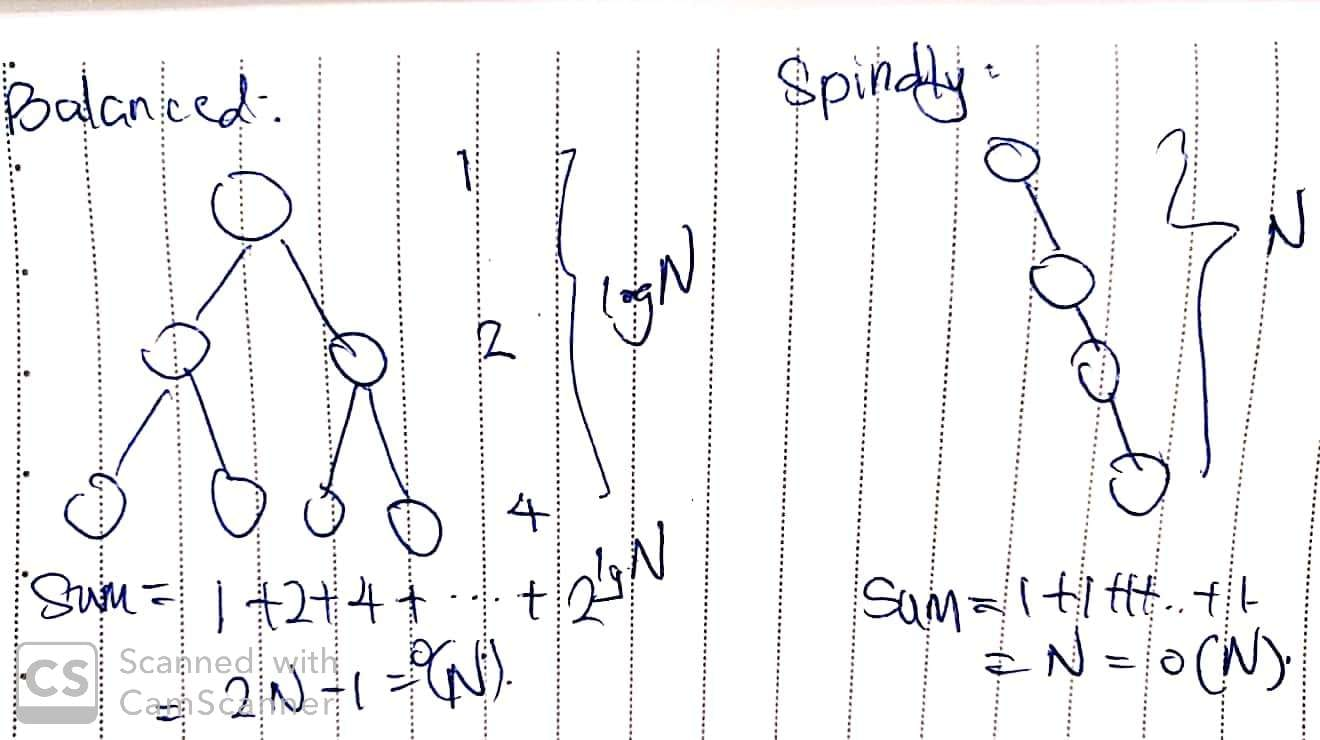# 104. Maximum Depth of Binary Tree

Reference: LeetCode
Difficulty: Easy

## Problem

Given a binary tree, find its maximum depth.

The maximum depth is the number of nodes along the longest path from the root node down to the farthest leaf node.

Note: A leaf is a node with no children.

Example:

Given binary tree [3,9,20,null,null,15,7]:

The return depth should be 3.

## Analysis

First, let’s be clear about the definition of height and depth of a tree or a node.

According to CS 61B, EPI p129, and a post on Stack Overflow:

• The height of a node is the maximum number of edges / links to any leaf.
• The height of a tree is the height of the root node.
• The depth of a node is the number of edges / links to the root.
• The maximum depth of a tree is equal to the height of the tree.However, according to the problem description, the maximum depth in this problem equals to the maximum number of nodes to any leaf. In other words, it is one plus our formal definition of height / maximum depth of a tree.

Methods:1. Recursion
• DFS (postorder) traverse the tree.
• Time: $O(N)$ since we visit each node exactly once no matter the tree is balanced or not.
• Space: $O(N)$ in the worst case; $O(\log{N})$ in the best case (balanced tree).
2. Tail Recursion + BFS
• The above recursion solution is probably not optimal in terms of space complexity. We can tweak the solution and make it tail recursion, so the compiler might optimize our code (reusing the same function call stack frame).
• To make it tail recursion, we just need to make sure that the recursive call is the last action in the function.
• Note: Optimization of tail recursion is not supported by Python or Java. And it is quite complicated.
• Note: A function cannot be tail recursion if there are multiple occurrences of recursive calls in the function, even if the last action is the recursive call, e.g. return f(n - 1) + f(n - 1).
3. Iteration
• With the help of a stack, we can simulate the function call process.
• Idea: Keep the next nodes to visit in a stack
• Time: $O(N)$
• Space: $O(N)$ in the worst case; $O(\log{N})$ in the best case (balanced tree).

## Code

Test Case:

### Iteration

Note:

• The initial depth should be 1.

CommentJunhao Wang
a software engineering cat